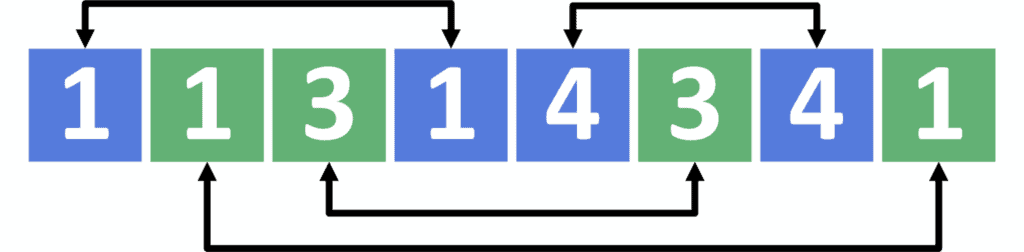## 1. Overview

In this tutorial, we’ll discuss the problem of pairing socks from an array that containspairs of socks.

First, we’ll define the problem and provide an example that explains it.

Second, we’ll present a naive approach. Then we’ll discuss two different approaches to improve it, and obtain better solutions.

## 2. Defining the Problem

Suppose we havepairs of socks randomly distributed inside an array of length. Each sock may have one or more specifications, such as size, color, etc; therefore, we have to pair each sock with another of the same specifications.

To understand this more clearly, let’s look at a simple example.

Let’s say that we have an array withsocks. Additionally, each sock has a special color and a special size:Now suppose that the color of each cell represents the color of the corresponding sock, while the number written inside the cell represents the sock size. Consequently, we can find the solution to pairing the socks:Note that in this tutorial, we’ll discuss finding only one possible solution; we won’t consider the case where we might have more than one answer.

## 3. Naive Approach

### 3.1. Main Idea

The main idea here is to iterate over the socks. For each sock, we’ll try to find a similar one that hasn’t been paired before.

### 3.2. Implementation

Let’s take a look at the implementation:Initially, we declare a list called, which will store the result as pairs of socks. In addition, we declare an array called, which expresses whether each sock has been taken before or not. Furthermore, we initialize this array withvalues.

Next we iterate over the socks. If the sock hasn’t been taken before, we try to find a similar one by iterating over the remaining socks and checking for similarities. If a match is found, we add the similar socks to, and flag both of them as taken.

Finally, we return, which stores the similar pairs of socks in the given array.

### 3.3. Complexity

The time complexity here is. Let’s examine the reason behind this complexity.

To start, we iterate over all the socks. For each one, we iterate over all the socks located after it inside the array. We’ll find that thesock will iterate oversocks because no socks come after it. Similarly, thesock will iterate oversock, and so on. As a result, this step will cost, which equals.

## 4. Sorting Approach

### 4.1. Main Idea

The main idea here is to sort the array of socks first. When we sort it, similar socks will be next to each other; therefore, we only have to iterate over the sorted array and check each sock with its adjacent.

### 4.2. Implementation

Let’s take a look at the implementation:Initially, we declare a list called, which will store the result as pairs of socks. Then we sort the given array and store it in.

Next we iterate over the socks, and check the similarity between thesock and theone. If they are similar, we add the similar socks to, and increase the iteratorbyto prevent the sock from joining more than one pair.

Finally, we return, which stores the similar pairs of socks in the given array.

### 4.3. Complexity

The time complexity here is. Let’s examine the reason behind this complexity.

The main complexity of this approach is the sorting algorithm, so we prefer to choose an algorithm withcomplexity as merge sort.

Then we iterate over all the socks once, and we only needto do that.

## 5. Hash Approach

### 5.1. Main Idea

The main idea here is to use a HashSet to store the last appearance of a similar sock. Consequently, we iterate over the socks, and in each step, we check whether the HashSet contains a similar sock or not.

### 5.2. Implementation

Let’s take a look at the implementation:Initially, we declare a list called, which will store the result as pairs of socks. We also declareas an empty HashSet.

Then we iterate over the socks, and check whethercontains a similar sock to theone. If it does, we add both thesock and the similar sock fromto the result. In addition, we delete the similar one to prevent the sock from joining more than one pair. If the HashSet doesn’t contain a similar sock, we add thesock to.

Finally, we return, which stores the similar pairs of socks in the given array.

### 5.3. Complexity

The time complexity here is.

First, we iterate over all the socks once, and we only needto do that. At each step, we check ifcontains a special sock, which has acomplexity. Also, the operation of adding and deleting fromhas acomplexity.

## 6. Conclusion

In this article, we presented the problem of pairing socks. We also explained the main ideas behind three different approaches to solving this problem, and walked through their implementations.